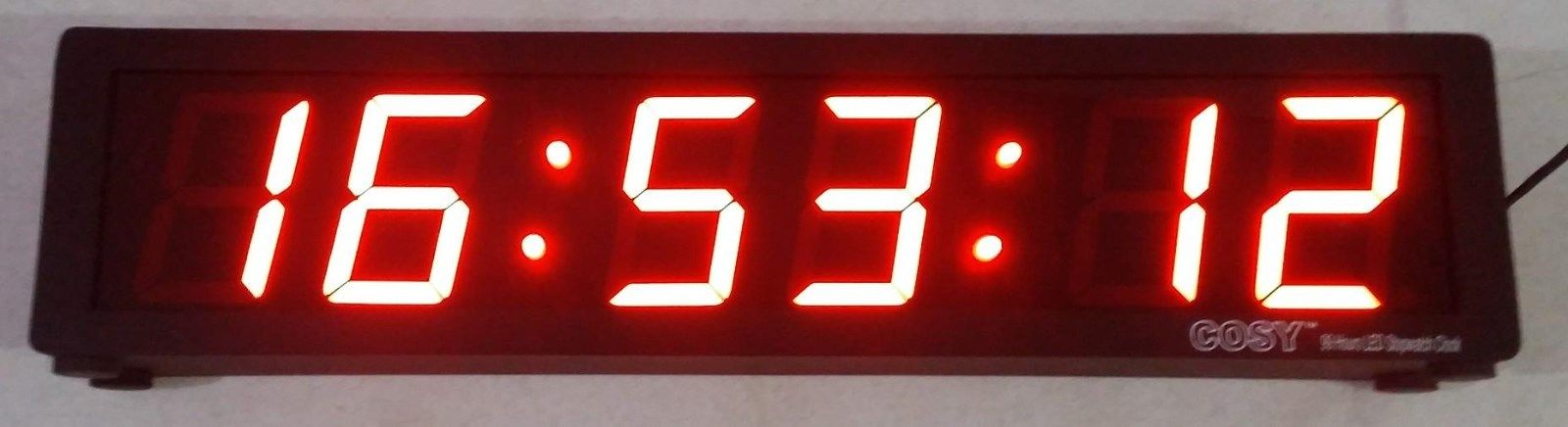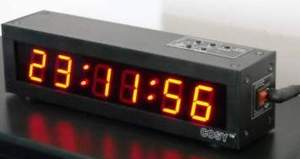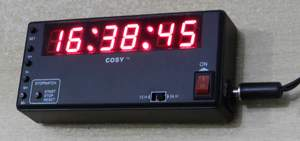What is the difference between Induction, Inductors & Inductance? Induction Current is produced in a conductor when it is moved through a magnetic field because the magnetic lines of force are applying a force on the free electrons in the conductor and causing them to move. This process of generating current in a conductor by placing the conductor in a changing magnetic field is called induction. Inductor: An electrical device (typically a conducting coil) that introduces inductance into a circuit. E.g choke Inductors introduce Inductance. Inductance The property of a circuit or circuit element that opposes a change in current flow, thus causing current changes to lag behind voltage changes. It is measured in henrys Mutual Inductance A measure of the relation between the change of current flow in one circuit to the electric potential generated in another by mutual induction. Like inductance, mutual inductance is measured in henries. Self Inductance: The property of an electric circuit or component that caused an emf to be generated in it as a result of a change in the current flowing through the circuit. Induced EMF : A voltage is induced when a conductor cuts magnetic field lines or when the magnetic field through a coil changes. This is called induced e.m.f. Impedance: The total opposition that a circuit offers to the flow of alternating current or any other varying current at a particular frequency. It is a combination of resistance R and reactance X, measured in ohms.VISIT ONLINE STORE FOR SHOPPINGEmail: vijayendra2@gmail.com

For more electronic kits visit: http://www.youtube.com/vijayendra2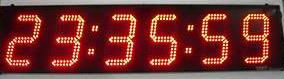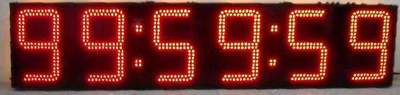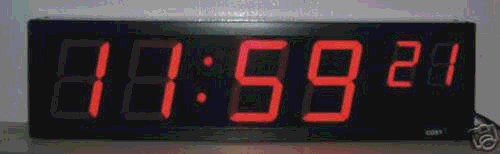STOPWATCHES